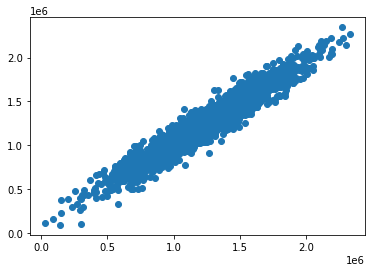A great simple example on how to deal with Linear Regression in Python utilizing Matplotlib, Seaborn, and Scikit-learn. The data is fake, only to be used as an example and found here.

# Linear Regression - Simple Example¶

### Utilizes a fake US Housing Dataset¶

In :
```import pandas as pd
import numpy as np
```
In :
```import matplotlib.pyplot as plt
import seaborn as sns
```
In :
```%matplotlib inline
```
In :
```#Fake data, but good for example
```
In :
```df.head()
```
Out:
Avg. Area Income Avg. Area House Age Avg. Area Number of Rooms Avg. Area Number of Bedrooms Area Population Price Address
0 79545.458574 5.682861 7.009188 4.09 23086.800503 1.059034e+06 208 Michael Ferry Apt. 674\nLaurabury, NE 3701...
1 79248.642455 6.002900 6.730821 3.09 40173.072174 1.505891e+06 188 Johnson Views Suite 079\nLake Kathleen, CA...
2 61287.067179 5.865890 8.512727 5.13 36882.159400 1.058988e+06 9127 Elizabeth Stravenue\nDanieltown, WI 06482...
3 63345.240046 7.188236 5.586729 3.26 34310.242831 1.260617e+06 USS Barnett\nFPO AP 44820
4 59982.197226 5.040555 7.839388 4.23 26354.109472 6.309435e+05 USNS Raymond\nFPO AE 09386
In :
```df.info()
```
```<class 'pandas.core.frame.DataFrame'>
RangeIndex: 5000 entries, 0 to 4999
Data columns (total 7 columns):
#   Column                        Non-Null Count  Dtype
---  ------                        --------------  -----
0   Avg. Area Income              5000 non-null   float64
1   Avg. Area House Age           5000 non-null   float64
2   Avg. Area Number of Rooms     5000 non-null   float64
3   Avg. Area Number of Bedrooms  5000 non-null   float64
4   Area Population               5000 non-null   float64
5   Price                         5000 non-null   float64
dtypes: float64(6), object(1)
memory usage: 273.6+ KB
```
In :
```df.describe()
```
Out:
Avg. Area Income Avg. Area House Age Avg. Area Number of Rooms Avg. Area Number of Bedrooms Area Population Price
count 5000.000000 5000.000000 5000.000000 5000.000000 5000.000000 5.000000e+03
mean 68583.108984 5.977222 6.987792 3.981330 36163.516039 1.232073e+06
std 10657.991214 0.991456 1.005833 1.234137 9925.650114 3.531176e+05
min 17796.631190 2.644304 3.236194 2.000000 172.610686 1.593866e+04
25% 61480.562388 5.322283 6.299250 3.140000 29403.928702 9.975771e+05
50% 68804.286404 5.970429 7.002902 4.050000 36199.406689 1.232669e+06
75% 75783.338666 6.650808 7.665871 4.490000 42861.290769 1.471210e+06
max 107701.748378 9.519088 10.759588 6.500000 69621.713378 2.469066e+06
In :
```df.columns
```
Out:
```Index(['Avg. Area Income', 'Avg. Area House Age', 'Avg. Area Number of Rooms',
'Avg. Area Number of Bedrooms', 'Area Population', 'Price', 'Address'],
dtype='object')```
In :
```X = df[['Avg. Area Income', 'Avg. Area House Age', 'Avg. Area Number of Rooms',
'Avg. Area Number of Bedrooms', 'Area Population']]
#Removed string column Address and Price since it's in the y
```
In :
```y = df['Price']
```
In :
```#Import sklearn toolkit
from sklearn.model_selection import train_test_split
```
In :
```#Choose the test size
#Test size = % of dataset allocated for testing (.4 = 40%)
#Random state = # of random splits
X_train, X_test, y_train, y_test = train_test_split(X, y, test_size=0.4, random_state=101)
```
In :
```from sklearn.linear_model import LinearRegression
```
In :
```#Linear Regression Obj
lm = LinearRegression()
```
In :
```#Fit
lm.fit(X_train,y_train)
```
Out:
`LinearRegression()`
In :
```#You can manually view coefficients before mapping to X
lm.coef_
```
Out:
```array([2.15282755e+01, 1.64883282e+05, 1.22368678e+05, 2.23380186e+03,
1.51504200e+01])```
In :
```#Create list of coefficients by X columns
cdf = pd.DataFrame(lm.coef_,X.columns,columns=['Coeff'])
```
In :
```cdf

#For every 1 unit increment in Avg. Area income, there is an approx. \$21 increase in price, etc.
```
Out:
Coeff
Avg. Area Income 21.528276
Avg. Area House Age 164883.282027
Avg. Area Number of Rooms 122368.678027
Avg. Area Number of Bedrooms 2233.801864
Area Population 15.150420

# Predictions¶

In :
```#Now let's try the testing set
predictions = lm.predict(X_test)
```
In :
```#Predicted prices of the homes
predictions
```
Out:
```array([1260960.70567627,  827588.75560334, 1742421.2425434 , ...,
372191.40626923, 1365217.15140897, 1914519.5417887 ])```
In :
```#Compare predicted prices of homes to actual
plt.scatter(y_test,predictions)
```
Out:
`<matplotlib.collections.PathCollection at 0x25e0c6a3808>`In :
```#Distribution of residuals
#Actual values of y_test vs. predicted
sns.distplot((y_test-predictions))
#Since it's normally distributed, this is good
```
Out:
`<matplotlib.axes._subplots.AxesSubplot at 0x25e0cac7308>`In :
```#Import metrics
from sklearn import metrics
```
In :
```#Get the mean absolute error
metrics.mean_absolute_error(y_test, predictions)
```
Out:
`82288.22251914948`
In :
```#Get the mean squared error
metrics.mean_squared_error(y_test, predictions)
```
Out:
`10460958907.209064`
In :
```#Get the root mean squared error
#One of the better metrics, most popular
#Essentially, the closer to 0 the better, but it depends in this case the avg home price.
#Our avg home price here is 1.2m, so this RMSE could be interpreted as good
np.sqrt(metrics.mean_squared_error(y_test, predictions))
```
Out:
`102278.8292229094`
In [ ]:
```#All done for now!
```